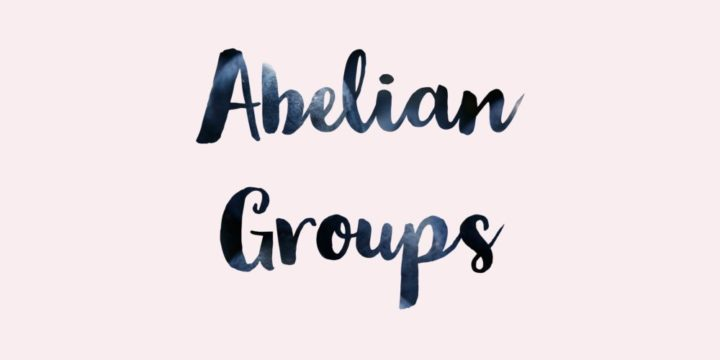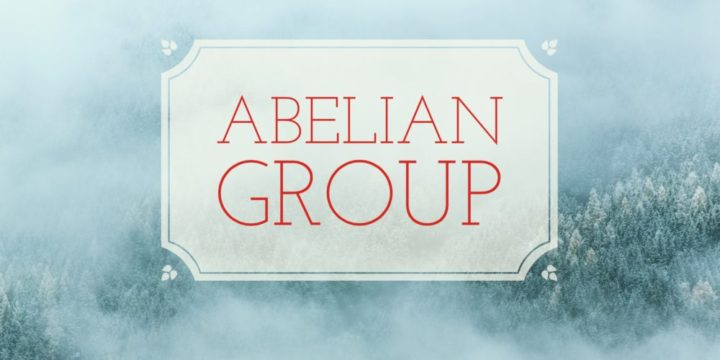# A Group Homomorphism and an Abelian Group## Problem 207

Let $G$ be a group. Define a map $f:G \to G$ by sending each element $g \in G$ to its inverse $g^{-1} \in G$.
Show that $G$ is an abelian group if and only if the map $f: G\to G$ is a group homomorphism.Add to solve later

## Proof.

### $(\implies)$ If $G$ is an abelian group, then $f$ is a homomorphism.

Suppose that $G$ is an abelian group. Then we have for any $g, h \in G$
\begin{align*}
f(gh)&=(gh)^{-1}=h^{-1}g^{-1}\\
&=g^{-1}h^{-1} \text{ since } G \text{ is abelian}\\
&=f(g)f(h).
\end{align*}
This implies that the map $f$ is a group homomorphism.

### $(\impliedby)$ If $f$ is a homomorphism, then $G$ is an abelian group.

Now we suppose that the map $f: G \to G$ is a group homomorphism.
Then for any $g, h \in G$, we have
$f(gh)=f(g)f(h) \tag{*}$ since $f$ is a group homomorphism.
The left hand side of (*) is
$f(gh)=(gh)^{-1}=h^{-1}g^{-1}.$ Thus we obtain from (*) that
$h^{-1}g^{-1}=g^{-1}h^{-1}.$ Taking the inverse of both sides, we have
$gh=hg$ for any $g, h \in G$.
It follows that $G$ is an abelian group.

## Related Question.

Another problem about the relation between an abelian group and a group homomorphism is:
A group is abelian if and only if squaring is a group homomorphismAdd to solve later

### 1 Response

1. 03/05/2017

[…] Another problem about the relation between an abelian group and a group homomorphism is: A group homomorphism and an abelian group. […]

This site uses Akismet to reduce spam. Learn how your comment data is processed.

###### More in Group Theory##### Order of the Product of Two Elements in an Abelian Group

Let $G$ be an abelian group with the identity element $1$. Let $a, b$ be elements of $G$ with order...

Close